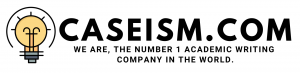# AMERICAN GREETINGS Case Solution & Answer

VALUATION OF THE BUSINESS THROUGH CASH FLOW ANALYSIS:

WACC Calculation:

The weighted average cost of calculation has two components, which are equity and debt. The cost of equity has been calculated through CAPM. The company is currently not seeking any expansion plan and its main emphasize is on the repurchase of stock. Therefore, the beta of the company has been taken which is equal to 1.63. The beta measures the risk position of the company in terms of its financial position.

The beta over 1 represents the company as more risky than the overall market. The risk free rate of the company is the 10 years Treasury bond rate that equals to 2.8%. The risk premium which is compensation to the investor to accept more risk is equal to 5%. The cost of equity is representing a figure of 10.95%.

Moreover, the after tax cost of debt has been calculated by using a formula â€œKd (1-T)â€, in which the cost of debt â€œkdâ€ is 5.8% as per the companyâ€™s bond rating of BB+ and tax rate is 40%. The after tax cost of debt is equal to 3.48%.

The market value of the debt is assumed to be equal to the book value, which is equal to \$235 million representing a weight age of 33% of the total capital. The market value of equity is calculated by taking a product of the â€œper share priceâ€ and â€œoutstanding sharesâ€ in year 2011, which is equal to \$479 million representing a weight age of 67% of the total capital. After all of these calculations, the WACC is calculated and equal to 8.5%. (These calculations referred to exhibit no. 1)

Valuation of Business in Bullish Trend:

The bullish trend shows the favorable position of the company despite of the overall industrial decline. In this case, the trend estimates that the operating margin, which is equal to the earnings before interest and tax, is equal to 9% of the sales revenue. The sales revenue will increase 3% in each year. The sales revenue has been calculated from this growth rate up to 5 years.

It is assumed that the amortization and depreciation expense is zero due to the limited availability of information. The working capital is also assumed to show an increase of 3%.

The NOPAT has been calculated using the tax rate of 40%.These are those profits that have an impact on the cash flows of the company. Beyond year 2015, the terminal value is calculated using the constant growth model with the assumed growth rate of 3%.

The next step is the calculation of net present value (Enterprise Value) using WACC and after this; the debt has been subtracted from this value to arrive at equity value. (These calculations referred to exhibit no. 2)

Bearish Trend:

Similar steps have been performed as in the valuation of the business in a bullish trend, except for the assumption derived in this case. The assumptions derived in this case are that the revenue of the company will not grow at all and the operating margin will show a decline representing 6% of the sales.

This means that the operating cost the company has been increased due to the immense competition and overall decline in the market. Furthermore, the working capital does not show the increase as a result of zero growth.

The Enterprise value in this case is lower than the above case. It can also be clearly judged due to the bearish trend. (These calculations referred to exhibit no. 3)

VALUATION THROUGH COMPARABLES:

Using Earning Yield Ratio:

As it is difficult to find the appropriate rival for the calculation of the earnings yield ratio, the average industry earnings yield ratio is taken to calculate the per share price of the company. The industry average shows a figure of 19%. Earning yield shows the amount earned by the company of the market price per share that is also known as return on equity via Market value.

Using EBIDTA Multiple:

The existing multiple provided in the case, which is equal to 3.5 times does not show an actual position of the company. This EBIDTA is currently showingthe internal position of the company. According to the trend of the market, which is calculated using the average of all these multiples, it shows that the level of EBIDTA must be higher than this and should at least represent a figure of 6.38 times. The market price per share using this multiple shows a relatively strong figure from the above method.(These calculations referred to exhibit no. 4& 5)

CONCLUSION & RECOMMENDATION:

The company is generating revenues with a decline. The company has adopted a strong branding strategy to get its market share. Its overall risk position as a result of its weighted average cost of capital shows acceptable position with a rate of less than 10%. The companyâ€™s has been evaluated using different methods with different scenarios.

The overall result shows that the price of that the company is currently undervalued in all of the methods except the earning yield method. The company should go for the share repurchase without any hesitation because it is an opportunity for the company to increase its return on equity. Moreover, it can raise further capital at higher rates in the future with a higher value as predicted by the models…………….

This is just a sample partial work. Please place the order on the website to get your own originally done case solution